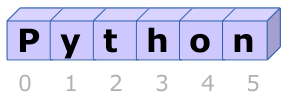# Strings

A string is a sequence of characters. For eg,

``````s = "Python"
``````

To access a single character, we can use a bracket operator and an index,

``````c1 = s
c2 = s
``````

`c1` stores `'P'` and `c2` stores `'y'`. Index starts from `0` for a `str` (or a list).

Indices must be integers. Try accessing a character using a non `int` index and observe the error.

Length starts counting from `1` not `0`. You can get the length of the string using `len` function.

``````len(s)
``````

It gives the answer as `6`.INSTRUCTIONS
• Define a function with the name `str_func` that takes one argument and returns the last character of the string passed to the function as a parameter.

Sample Input:
`str_func('1234')`
Sample Output:
`'4'`

• Define another function with the name `sum_str_func` that takes one argument and returns the sum of all digits in that number. The argument passed can be `str` or an `int`. You need to convert the integer to a string to calculate it's length, and then use a loop to add each digit to calculate the sum of all digits.

Sample Input:
`sum_str_func('1234')`
Sample Output:
`10`

No hints are availble for this assesment

Answer is not availble for this assesment

Note - Having trouble with the assessment engine? Follow the steps listed here